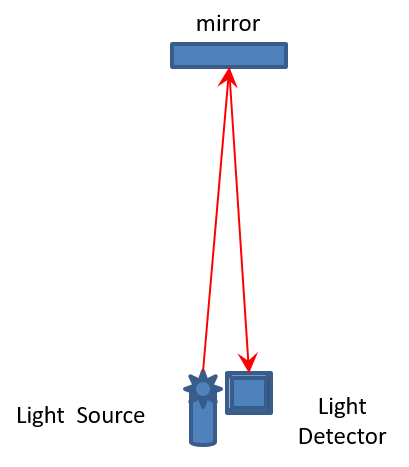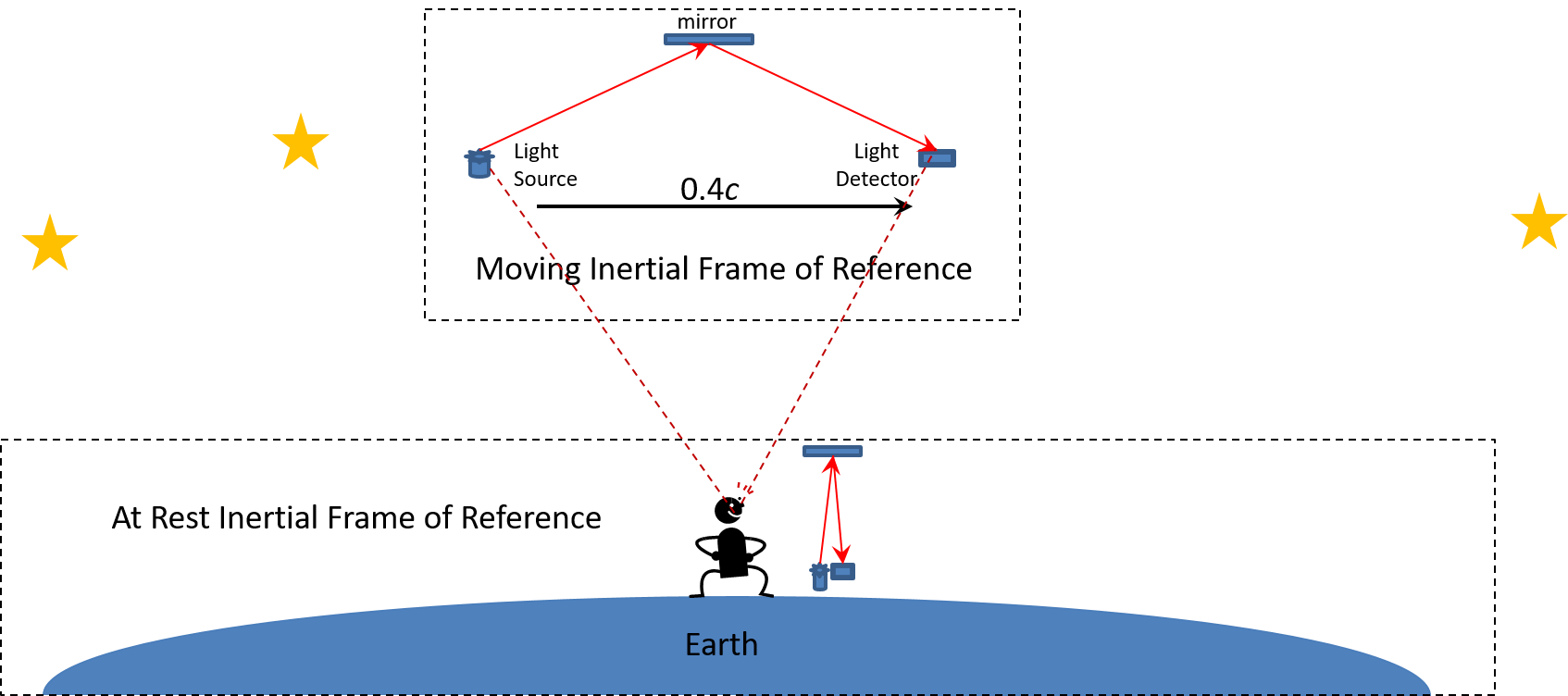# Speed of Light

The second assumption made by Albert Einstein in his Special Theory of Relativity was the Principle of Invariant Light Speed. This principle states that the speed of light in the vacuum of space is the value c, independent of its source and in all frames of reference. The constant c is considered a universal constant with the value 299,792,458 m/s. Many scientific experiments have confirmed this value within an Inertial Frame of Reference. The Apollo 11 astronauts left a special mirror on the moon that reflects any light aimed at the mirror back in a parallel line to its source. This mirror can be used by any Earth-based lasers to verify the constant c.

In another one of Einstein’s thought experiments, Einstein imagined a light clock. A light clock is illustrated in the following figure:A light clock consists of a light source, a mirror, and a light detector. Included in the mechanism is a clock that determines the amount of time it takes for a photon emitted by the light source to bounce off the mirror and return to the light detector. Knowing the distance of the mirror from the light source, the light clock will always measure the speed of the photon as the constant c in the vacuum of space.

A light clock is one of the experiments that can be performed within any Inertial Frame of Reference as illustrated below:In the At Rest Inertial Frame of Reference, as well as all the Moving Inertial Frames of Reference, the local observer within each frame of reference will measure the speed of light as exactly c. While Einstein assumed the speed of light was independent of its source, these same results would be achieved if the speed of light was dependent on its local light source.

To understand the difference between “independent” and “dependent” of a light source, one must look at how the observer on Earth would measure the speed of a photon generated from a light source within a Moving Inertial Frame of Reference. This situation is illustrated below:From the perspective of the observer on Earth (i.e. the At Rest Inertial Frame of Reference), the photon in the Moving Inertial Frame of Reference would travel a greater distance. This happens because the light source is in a Moving Frame of Reference traveling at a velocity of 40% of the speed of light relative to the At Rest Inertial Frame of Reference.

According to Einstein, the photon travels at speed c independent of its light source. This means the photons in the light clocks of the At Rest Inertial Frame of Reference and the Moving Inertial Frame of Reference are both travelling at speed c. However, the distance the photons travel in the two light clocks is considerably different. Einstein argued that this difference in length was due to time dilation. Moving clocks run slower. Since the moving frame of reference is travelling much faster than the at rest frame of reference, the clock in the moving frame of reference is running much slower. The slower clock causes the photon to appear to travel a much farther distance in the moving frame of reference.

In an alternative assumption, the photon travels at speed c dependent (or relative to) its light source. In this case, the photon in the Moving Inertial Frame of Reference is viewed by the observer on Earth as travelling at 1.4 times speed c. The photon in the moving frame of reference is traveling at speed c relative to its local light source, but the light source in the moving frame of reference is traveling 0.4c relative to the at rest frame of reference. These two relative speeds add to a speed of 1.4c for the photon in the moving frame of reference relative to the at rest frame of reference. Since the photon is travelling much faster in the moving frame of reference, it is observed moving a much greater distance than a photon being emitted in the local at rest frame of reference.

Keep in mind the concepts of photons moving at a speed independent or dependent on the speed of the light source are both assumptions. Since light travels in all directions from a light source, photons from the Moving Inertial Frame of Reference can arrive and be detected within Earth’s At Rest Inertial Frame of Reference. To determine whether the photon from the moving frame of reference is moving at speed c or 1.4c, all we need to do is measure the speed of the photon as it arrives on Earth. There are three techniques commonly used to measure the velocity of an object. These are as follows:

1.       Use a radar to bounce an electromagnetic signal off the photon like a radar gun. The problem with this approach is the Pauli Exclusion Principle. A photon is not solid, and the electromagnetic signal would pass right through the photon.

2.       Detect the photon and measure its momentum. Now we run into the Heisenberg Uncertainty Principle that states we cannot know both the location and the momentum of a photon at the same time. When we detect a photon, we cannot determine its momentum.

3.       Detect the same photon at two different locations along its path and measure the time it takes for the photon to travel the known distance between the two points. The problem here is that the detection of the photon also destroys the photon. One could argue that, once a photon is detected, another photon is emitted to continue the journey. The problem here is that the newly emitted photon is re-emitted within the At Rest Inertial Frame of Reference. This means it is re-emitted at speed c and not the original speed of the photon coming from the Moving Inertial Frame of Reference. As a result, when the newly emitted photon reaches the second location, it will always measure a speed of c regardless of the speed of the original photon.The bottom line here is that one cannot determine the speed of a random photon arriving at Earth from another Moving Inertial Frame of Reference. Hence, one cannot prove whether photons travel at speeds independent of their source as Einstein assumes, or whether photons travel at speeds dependent on their source. Both assumptions have an equal chance of being the correct assumption.

There are other indicators that photons travel at non-constant speeds. We know from the field of optics that light travels at different speeds through different mediums. We also know that rainbows are caused by different wavelengths of light travelling at different speeds through a raindrop and being deflected at different angles as a result. It is these issues that caused Einstein to limit his Principle of Invariant Light Speed to the vacuum of space.

However, even within the vacuum of space, there are issues regarding a constant speed of light. Due to Einstein’s Mass-Equivalence Equation, E=mc2, we know that photons have a mass equivalency. This mass equivalency is the reason astronomers can see objects that exist behind another object of great mass. The paths of photons are bent by the gravity of the massive object. If the path of a photon can be bent by gravitational forces, then photons can also be accelerated and decelerated by those same gravitational forces. Black Holes seemingly can stop photons altogether from escaping the gravitational pull of the Black Hole.

Of course, Einstein’s Theory of General Relativity always argues that gravitational time dilation is at play when photons encounter gravitational forces. The gravitational forces of Black Holes are so intense that they cause time to stand still. Einstein’s assumption of the Principle of Invariant Light Speed is hard to accept because it does not seem to logically follow our intuitive sense of physics. In our high school Physics courses, gravity causes objects to accelerate as they fall to Earth. Why are photon’s different?

The fact the world’s scientists base their research on Einstein’s Theory of Relativity is the basis for the Romeo Effect. Just as Romeo committed suicide based on the assumption that Juliet was deceased, our scientists are basing their new theories on the assumption that Einstein was correct in assuming the Principle of Invariant Light Speed. If we assume the speed of light in a vacuum is non-constant, then a new set of theories can be produced that are more logical in nature.

The Romeo Effect series of books use the assumption that the initial speed of photons is c, dependent on the speed of the light source, and that gravity changes the speed of photons due to the mass equivalency of the photons.